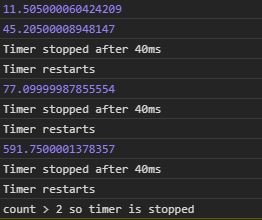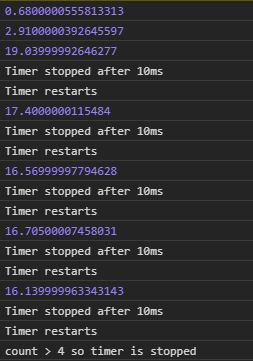# D3.js timer.restart() Function

• Last Updated : 29 Jul, 2020

The timer.restart() function in D3.js is used to restart a timer with the given function and delay. The timer.restart() function is used when one wants to reset the timer and start again.

Syntax:

Hey geek! The constant emerging technologies in the world of web development always keeps the excitement for this subject through the roof. But before you tackle the big projects, we suggest you start by learning the basics. Kickstart your web development journey by learning JS concepts with our JavaScript Course. Now at it's lowest price ever!

```timer.restart(callback, delay);
```

Parameters: It takes two parameters as mentioned above and described below:

• callback: It is the function to be stopped or start after a particular delay.
• delay: It is the time after which the function will be executed or stop

Example 1: When no delay is given.

## HTML

 ```<``html` `lang``=``"en"``>`` ` `<``head``>``    ``<``meta` `charset``=``"UTF-8"``>``    ``<``meta` `name``=``"viewport"` `content``=``        ``"width=device-width, initial-scale=1.0"``>```` ` `<``body``>``    ````    ``<``script` `type``=``"text/javascript"` `        ``src``=``"https://d3js.org/d3.v4.min.js"``>``    ````     ` `    ``<``script``>``        ``count = 0;``        ``let func = function (e) {``            ``console.log(e)``            ``if (e > 40) {``                ``console.log("Timer stopped after 40ms")``                ``if (e > 40) {``                    ``count++;`` ` `                    ``// Restarting the timer again``                    ``console.log("Timer restarts")``                    ``timer.restart(func)``                ``}``                ``if (count > 2) {``                    ``timer.stop();``                    ``console.log(``                        ``"count > 2 so timer is stopped")``                ``}``            ``}``        ``}``        ``var timer = d3.timer(func);``    `````` ` ``

Output:Example 2: When a delay is given.

## HTML

 ```<``html` `lang``=``"en"``>`` ` `<``head``>``    ``<``meta` `charset``=``"UTF-8"``>``    ``<``meta` `name``=``"viewport"` `content``=``        ``"width=device-width, initial-scale=1.0"``>```` ` `<``body``>``    ````    ``<``script` `type``=``"text/javascript"` `        ``src``=``"https://d3js.org/d3.v4.min.js"``>``    ````     ` `    ``<``script``>``        ``count = 0;``        ``let func = function (e) {``            ``console.log(e)``            ``if (e > 10) {``                ``console.log("Timer stopped after 10ms")``                ``if (e > 10) {``                    ``count++;`` ` `                    ``// Restarting the timer again``                    ``console.log("Timer restarts")``                    ``timer.restart(func)``                ``}``                ``if (count > 4) {``                    ``timer.stop();``                    ``console.log(``                    ``"count > 4 so timer is stopped")``                ``}``            ``}``        ``}``        ``// A delay of 2000ms``        ``var timer = d3.timer(func, 2000);``    `````` ` ``

Output:My Personal Notes arrow_drop_up